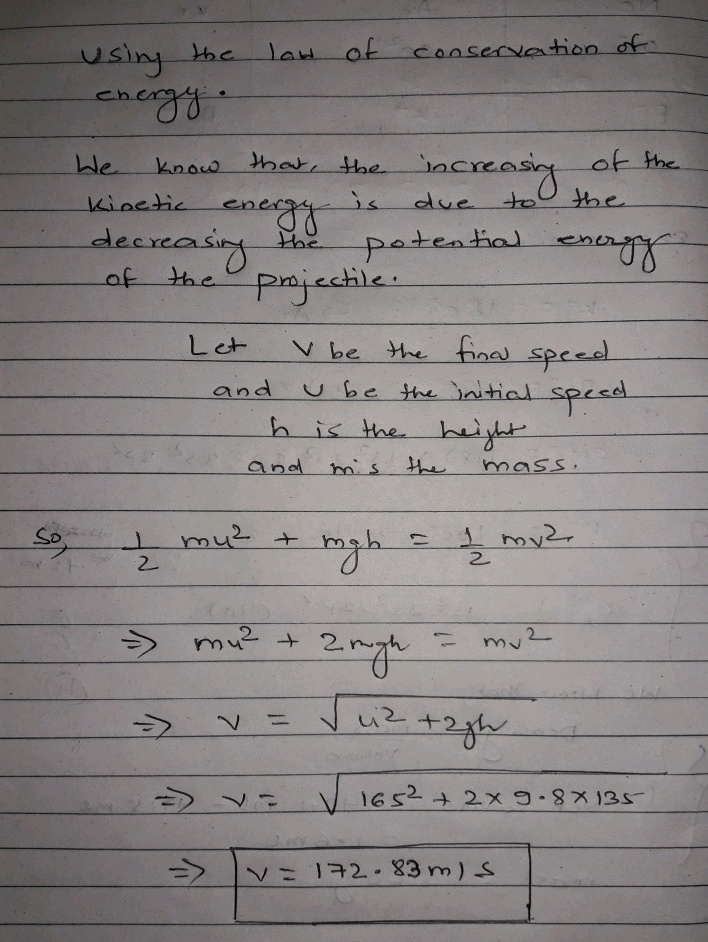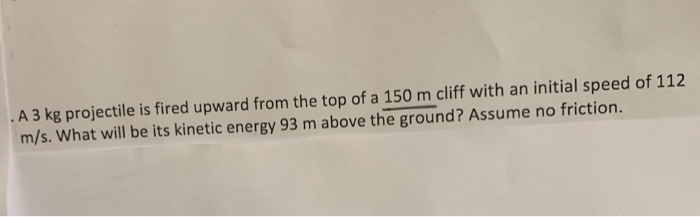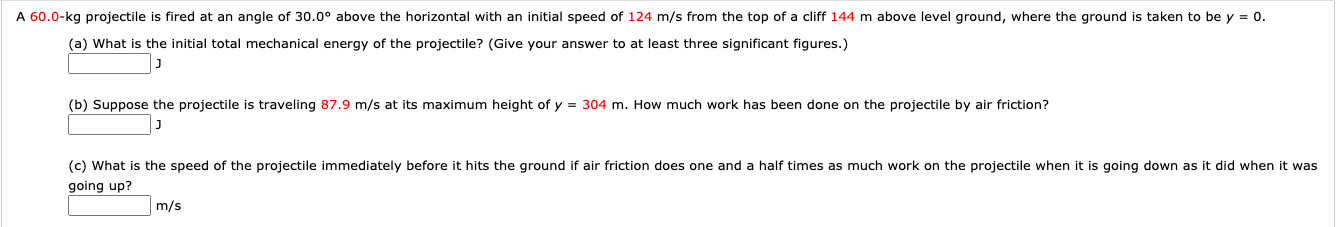# A projectile is fired at an upward angle of 38.0 degrees from the top of a...

A projectile is fired at an upward angle of 38.0 degrees from the top of a 135m high cluff with a speed of 165 m/s. What will be its speed when it strikes the ground below? (Use conservation of energy)

I would really appreciate a thorough walkthrough of this problem, thank you!#### Earn Coin

Coins can be redeemed for fabulous gifts.

Similar Homework Help Questions
• ### A projectile is fired at an upward angle of 55.0 degrees from the top of a 230m cliff with a speed of 180m/s

A projectile is fired at an upward angle of 55.0 degrees from the top of a 230m cliff with a speed of 180m/s.What will be its speed when it strikes the ground below? (Use conservation of energy.)

• ### projectile fired at an upward angle

A projectile is fired at an upward angle of 50.0 from the top of a 215-cliff with a speed of 145 .What will be its speed when it strikes the ground below? (Use conservation of energy.)

• ### A 0.2 kg cannon ball is fired at an upward angle of 45° from the top...

A 0.2 kg cannon ball is fired at an upward angle of 45° from the top of a 165 m cliff with a speed of 175 m/s. (A) Using conservation of energy, find the speed it has when it strikes the ground below. Suppose the cannon ball lands in a soft, muddy field. The force of the field is 75 N on the projectile. (B) How deep does it penetrate into the ground? -------------------------------------------------------------------------------- How much energy does it take...

• ### A ball is launched at an upward angle of 45 degrees from the top of a cliff 165 meters high, with a speed of 180 m/s

A ball is launched at an upward angle of 45 degrees from the top of a cliff 165 meters high, with a speed of 180 m/s. Use conservation of energy to find how fast it will be traveling when it hits the ground.

• ### A projectile is fired from ground level with a speed of 40 m/s at an angle of 30 degrees and hits a building 100m away

A projectile is fired from ground level with a speed of 40 m/s at an angle of 30 degrees and hits a building 100m away. What is the time of flight of the projectile? How high above ground does the projectile hit the building? What is the speed of the projectile just before it strikes the building?

• ### . A 3 kg projectile is fired upward from the top of a 150 m cliff.... A 3 kg projectile is fired upward from the top of a 150 m cliff with an initial speed of 112 m/s.What will be its kinetic energy 93 m above the ground? Assume no friction.

• ### An M-16 is fired from level ground at an upwards angle of 10 degrees with respect...

An M-16 is fired from level ground at an upwards angle of 10 degrees with respect to the horizontal. The initial velocity of the bullet is 948 m/s, but because it is fired at a 10 degree angle, the vertical component of the initial velocity is 165 m/s and the horizontal component is 934 m/s. Ignore air resistance and the curvature of the earth in this problem. (Hint: See the videos Projectile Motion Parts 9-13. For questions 9-14 and 17,...

• ### A projectile is fired from ground level at an angle of 40 degrees with muzzle speed...

A projectile is fired from ground level at an angle of 40 degrees with muzzle speed 110 ft/sec. Find the time of flight and the range.

• ### A 44.0-kg projectile is fired at an angle of 30.0° above the horizontal with an initial speed...

A 44.0-kg projectile is fired at an angle of 30.0° above the horizontal with an initial speed of 1.24 102 m/s from the top of a cliff 130 m above level ground, wherethe ground is taken to be y = 0.(a) What is the initial total mechanical energy of the projectile?(b) Suppose the projectile is traveling 87.9 m/s at its maximum height of y = 290 m. How much work has been done on the projectile by air friction?(c) What...

• ### A 60.0-kg projectile is fired at an angle of 30.0° above the horizontal with an initial...A 60.0-kg projectile is fired at an angle of 30.0° above the horizontal with an initial speed of 124 m/s from the top of a cliff 144 m above level ground, where the ground is taken to be y = 0. (a) What is the initial total mechanical energy of the projectile? (Give your answer to at least three significant figures.) (b) Suppose the projectile is traveling 87.9 m/s at its maximum height of y = 304 m. How much...

Free Homework App